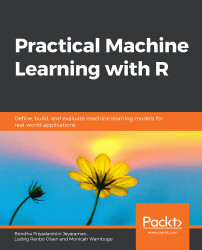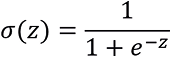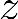•#### Practical Machine Learning with R#### Overview of this book

With huge amounts of data being generated every moment, businesses need applications that apply complex mathematical calculations to data repeatedly and at speed. With machine learning techniques and R, you can easily develop these kinds of applications in an efficient way. Practical Machine Learning with R begins by helping you grasp the basics of machine learning methods, while also highlighting how and why they work. You will understand how to get these algorithms to work in practice, rather than focusing on mathematical derivations. As you progress from one chapter to another, you will gain hands-on experience of building a machine learning solution in R. Next, using R packages such as rpart, random forest, and multiple imputation by chained equations (MICE), you will learn to implement algorithms including neural net classifier, decision trees, and linear and non-linear regression. As you progress through the book, you’ll delve into various machine learning techniques for both supervised and unsupervised learning approaches. In addition to this, you’ll gain insights into partitioning the datasets and mechanisms to evaluate the results from each model and be able to compare them. By the end of this book, you will have gained expertise in solving your business problems, starting by forming a good problem statement, selecting the most appropriate model to solve your problem, and then ensuring that you do not overtrain it.Free Chapter
An Introduction to Machine LearningData Cleaning and Pre-processingFeature EngineeringIntroduction to neuralnet and Evaluation MethodsLinear and Logistic Regression ModelsUnsupervised LearningAppendix## Logistic Regression

In linear regression, we modeled continuous values, such as the price of a home. In (binomial) logistic regression, we apply a logistic sigmoid function to the output, resulting in a value between 0 and 1. This value can be interpreted as the probability that the observation belongs to class 1. By setting a cutoff/threshold (such as 0.5), we can use it as a classifier. This is the same approach we used with the neural networks in the previous chapter. The sigmoid function is, whereis the output from the linear regression:

###### Figure 5.21: A plot of the sigmoid function

Figure 5.21 shows the sigmoid function applied to the output. The dashed line represents our cutoff of 0.5. If the predicted probability is above this line, the observation is predicted to be in class 1, otherwise, it's in class 0.

For logistic regression, we use the generalized version of lm(), called glm(), which can be used for multiple types of regression. As we are performing binary...